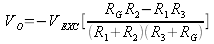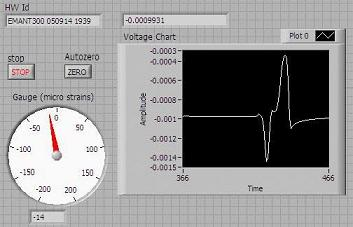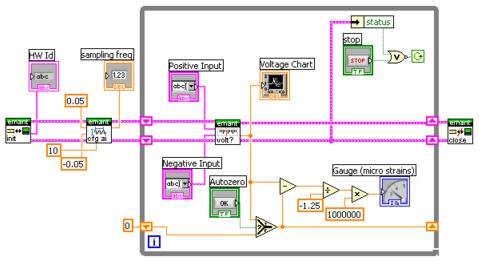# Strain Gauge (Gage) Data Acquisition

When used with a notebook, the EMANT300 USB 24 bit (DAQ) Data Acquisition module becomes a low cost portable, battery-operated instrument which can be configured as a six channel Strain Indicator and Recorder .

When an object is stretched due to an external force and the length of the object increases from L to L+ΔL, the ratio ΔL/L is called strain.

ε = ΔL/L

As the ratio of deformation is often very small, it is often represented in a units of 10-6 or μstrainA strain gauge (gage) can be used to measure the strain of this object. The most common type of strain gauge (gage) consists of a flexible backing which supports a metallic foil pattern etched onto the backing. As the object is deformed, the foil pattern is deformed, causing its electrical resistance to change. This resistance change, usually measured using a Wheatstone bridge circuit, can be used to calculate the exact amount of deformation by means of the quantity known as the gauge (gage) factor.

The gauge(gage) factor of a strain gauge (gage) relates strain to change in electrical resistance. The gauge (gage) factor GF is defined by the formulawhere RG is the resistance of the undeformed gauge, ΔR is the change in resistance caused by strain, and ε is strain.

In our example, we will use one strain gauge (gage) with GF=2, RG = 120 ohms and connected in a quarter bridge configuration. The bridge is excited at VEXC=2.5V. As the current required at the bridge is much higher than what is available from REFOUT, a simple buffer amplifier is required. If you prefer not to build your own circuit, you can use our Bridge Sensor Application Adaptor.The voltage output of the wheatstone bridge VO (seen at the differential input AIN1, AIN0) is given byIf the bridge is balanced VO =0 since all the resistances are equal. When a strain is applied, RG becomes R + ΔR and substituting R to all the other resistances, the equation becomesIf we assume 2 ΔR << 4RFinally substituting VEXC=2.5V, GF=2, we obtain

ε = ΔVO / 1.25

The LabVIEW VI that reads the voltage across the bridge, converts the voltage to μstrain is called EMANT300 Example Strain Gauge.VI Click here to watch the Strain Measurement Video

## Strain Gage LabVIEW Front Panel## Strain Gage LabVIEW Block Diagram